Model Name Baume: Valid for SRD

`1. What is does?`

Converts line density and temperature into ‘Baume’ unit, a method of measurement of a chemical’s specific gravity using hydrometers.

`2. Application`

Sugar beet processing, ophthalmics, starch industry, winemaking, industrial water treatment, and printed circuit board (PCB) fabrication.

`3. Maths`

Below is found the mathematical formula for Baume's degree calculation.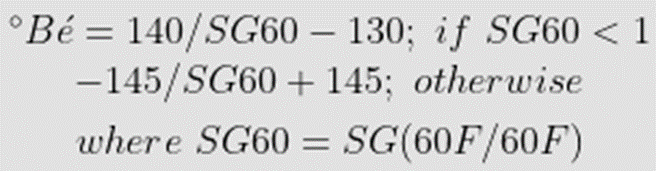To obtain the correct measurements for the Baume degree, the SRD and SMET work together as a powerful tool for measuring density, which is an extremely significant variable. The user would need to input:

3.1. Dataset of densities and temperatures.

3.2. Input Water density at T2 (60°F – Baume). Needed for Specific Gravity @60°F calculation. Compensated density is calculated with the values measured with SMET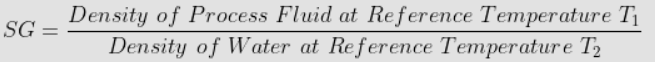3.3. Convert measure line density and line temperatures. Values are taken from SMET-SRD.

3.4. Convert the line density of process fluid to temperature compensated for density value at T1 (60°F –Baume) – By using a dataset table from a known product.

`4. How to load models into the SMET?`

The calculation tab allows configuring the SMET to run mathematical models for Viscosity, Density, and Concentration.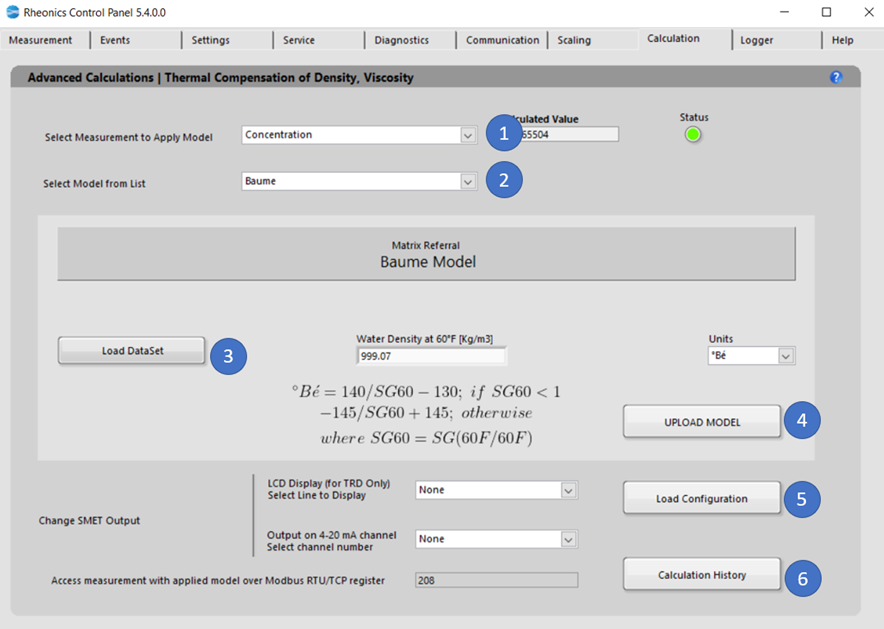Figure 1. Calculation tab for Brix degree model

The following steps are to load the models into SMET:

1.    In the Dropdown list “Select Measurement to Apply Model” select. That will modify the “Select Model from List” dropdown menu values. Select Measurement application (Concentration)

2.    In “Select Model from list” select the model you would like to use for the specific parameter. When you select the model, you will get more information about the specific equation used and the coefficients you will need to apply to that model.  Select “Baume”

3.    “Load Dataset” button, this operation is crucial to get the correct measurement for Baume degree so that density and temperature to be measured from the process must be within this table. Dataset table must cover at least the following instances:

a.    Five reference temperatures (at least)

b.    The density for each of four product types at the five reference temperatures from Process (60 reference points in all, at least)

c.    The base temperature(60°F), must be one of the five reference temperatures.

d.    Guarantee inline density value is between the density values from the table otherwise output from SMET will be NaN.

`5. Matrix Referral`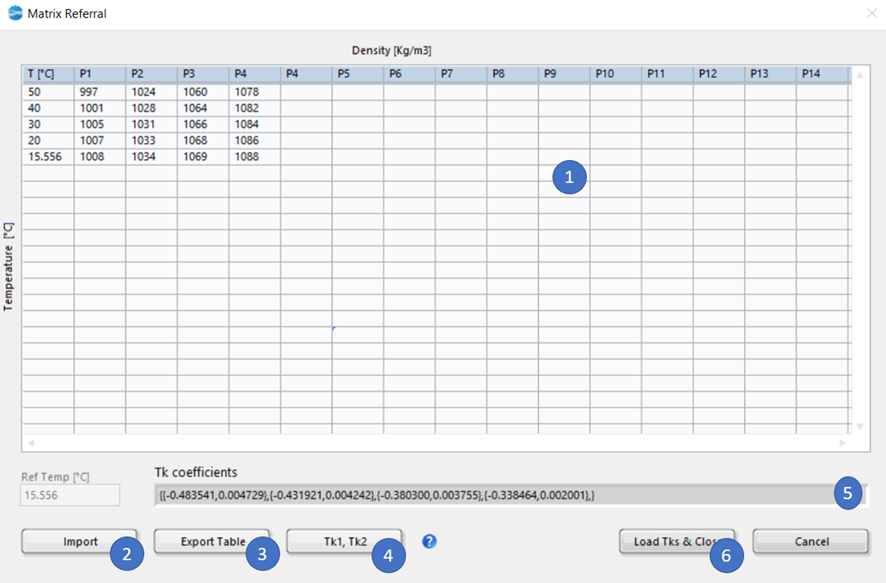Figure 2. Sample Dataset for Ferric Chloride (input values is Celcious instead Farenheit).

5.1. Dataset for Ferric Chloride, first column is for temperature. The rest of the columns should be each for a product "P", and the densities of that product at the different temperatures.

5.2. Import: Select dataset from an .csv sheet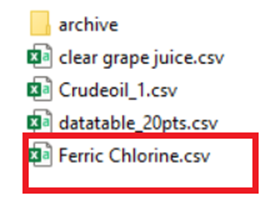Figure 4. Selecting dataset

5.3. Export: Export current table to .csv

5.4. "Tk1, Tk2" button to create the coefficients that will be used on the model.

5.5. Calculated Tk coefficients by internal model.

5.6. "Load Tks & Close" button to load these coefficients into the model

`6. Uploading the Mathematical Model`

Click “Upload Model” to load the model settings into SMET. The button should turn GREEN for a couple of seconds if the action was successful or turn RED if the operation was Rheonics Control Panel Software unsuccessful. This action will refresh the values for the Uncompensated and Compensated indicators. Here you can verify if the model is calculating the expected values. If not, you can correct and repeat the process.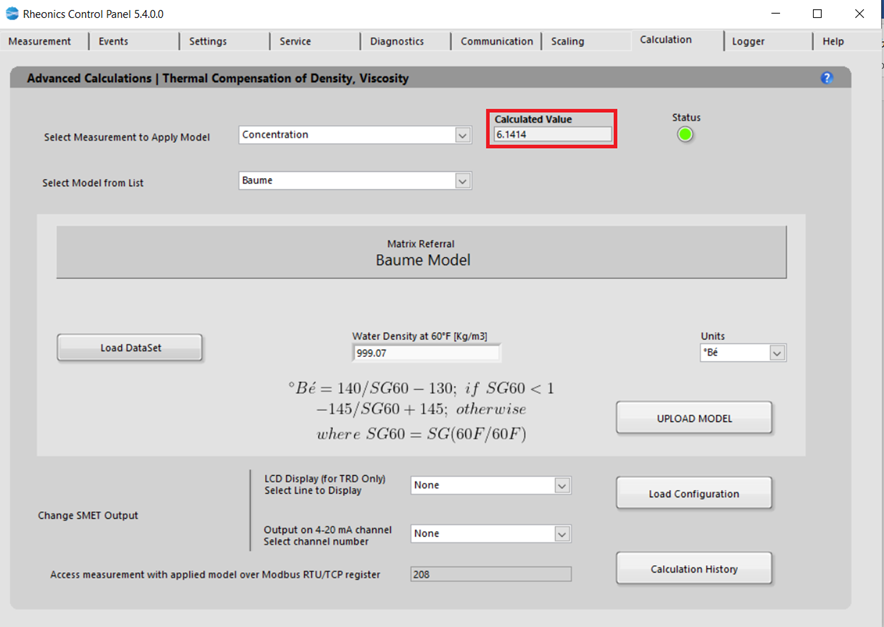Figure 4. Baume model loaded into the SMET.

6.1. Click “Load Configuration” to update the display and channel configurations in SMET.

• LCD Display

Select the line to display the parameter: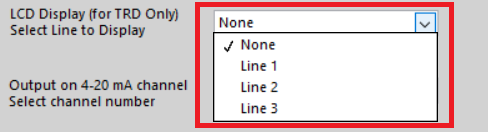Figure 5. Selecting read line for LCD display

Changes can be verified on the “Communication” Tab

• Analog outputs

Select analog channel to send data, this can be verified in “Service” TabFigure 6. Selecting analog output channel

• Modbus TCP/IP register

Measurement can always be accessed at the register address that is displayed.

• “Calculation History” button allows you to open a file where the last uploaded models and coefficients for these models are stored. This is for user reference.